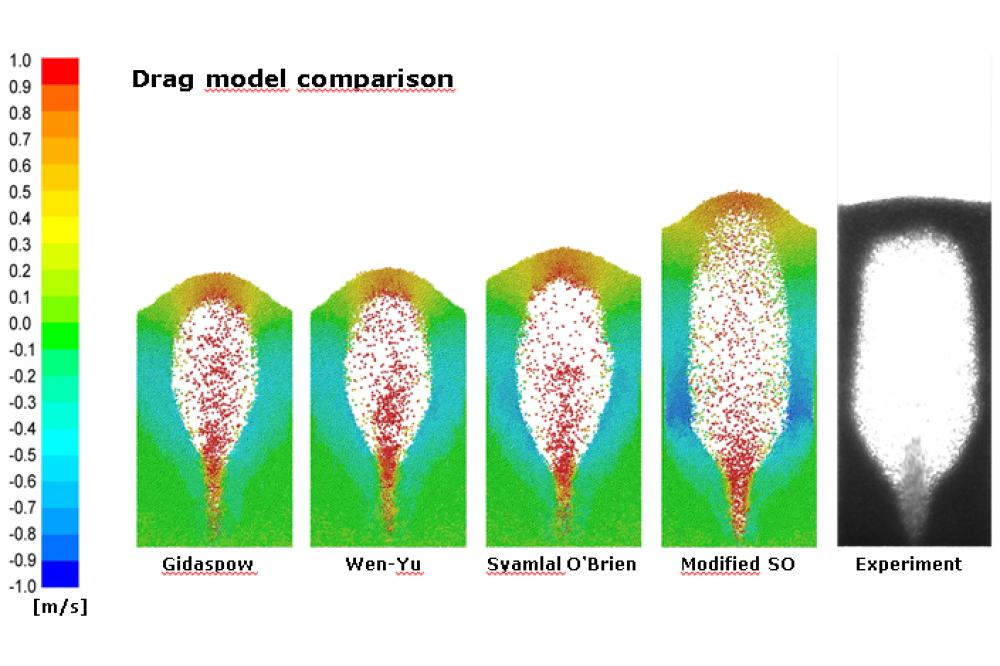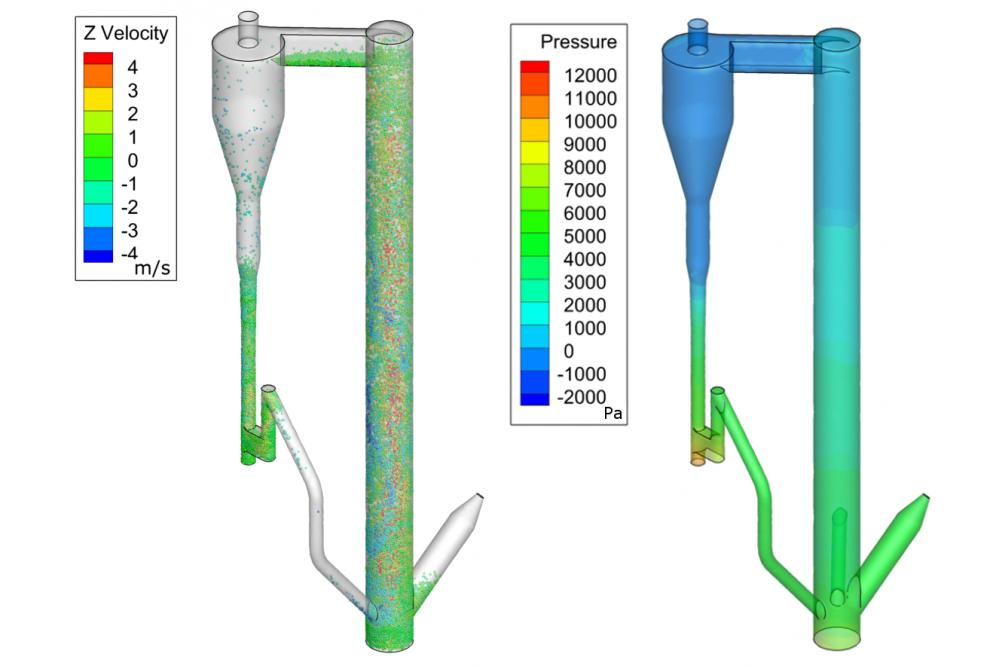# Scale-up of Calcium Carbonate Looping Technology for Efficient CO2 Capture from Power and Industrial PlantsEfficient CO2 Capture Abb.1

Fig. 1: Z-velocity snapshots at 250 ms

A. StrohEfficient CO2 Capture Abb.2

Fig. 2: Simulation of 1 MWth fluidized bed reactor with DDPM approach

A. Stroh

## Introduction

Multiphase flows are widely present in chemical processes, energy supply and carbon capture and storage technologies (CCS). In order to understand the hydrodynamics of particle transport and segregation phenomena of multiphase flows, computational fluid dynamics (CFD) simulations are applied. Basically, one can distinguish between the Euler-Euler approach and Euler-Lagrangian approach to calculate the flow-field, the interaction terms within one phase and between phases.

## Methods

The latter approach is usually quoted in the literature as the discrete particle model (DPM) for systems with low particle loading (particle volume fraction αp < ≈ 10%) and dense discrete particle model (DDPM) for dense particle systems (αp > ≈ 60%). In the discrete phase model the particle trajectories are calculated for each particle, which makes this approach computational expensive for particle systems with more than 100,000 particles. In order to simulate particle systems with millions of particles, a simplification is made where thousands of particles are modelled in so called parcels. In this approach one parcel represents several thousands of particles. The collision forces between parcels take into account the total mass and volume of their represented particles. This simplification overcomes endless computational time following the trajectories of millions of particles.

## Results

The following results were produced with the software ANSYS FLUENT using the DPM model. Figure 1 depicts simulated snapshots of the velocity field at 250 ms and shows the influence of different drag models on the particle velocity. In this simple case of a cold flow experiment every parcel represents one particle. In total 36,000 thousand of particles with a diameter of 2.5 mm were simulated. The required computational time for conducting a transient simulation of 500 ms total time was approximately 24 hours, with 4 parallel cores of 3.5 GHz each. Obviously the drag model has a crucial role in predicting the flow pattern of the particles. Thus one focus of research will remain on the development of reliable drag models for different particle shapes and diameter ranges. In Figure 2 a snapshot of the velocity and pressure field of a circulating fluidized bed reactor (CFB) is presented.

## Discussion

The pressure along the height of the riser is decreasing which is in agreement with experimental observations. These results fully confirm the great advantage of numerical simulation tools, accessing difficult measureable process parameter such as solid mass flow. The simulation was performed considering ≈ 44.000 parcels and a total bed inventory of 260 kg. The simulation of 10 s real time required 1 week of computational time with 16 parallelized cores.

## Outlook

In the further work, combustion reactions will be implemented in the numerical model in order to investigate the influence of energy exchange between the two interacting phases.

## Last Update

• Last Update: 2020-11-12 18:35